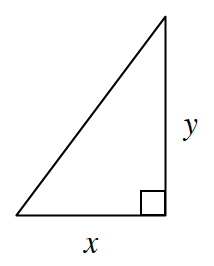### Home > CCG > Chapter 10 > Lesson 10.2.1 > Problem10-72

10-72.

Multiple Choice: Which expression below represents the length of the hypotenuse of the triangle at right?

1. $\frac { y } { x }$

1. $\sqrt { x ^ { 2 } + y ^ { 2 } }$

1. $x + y$

1. $\sqrt { y ^ { 2 } - x ^ { 2 } }$

1. None of theseIf $c =\text{ hypotenuse}$, $c^2 = x^2 + y^2$. Solve for $c$.

(b)